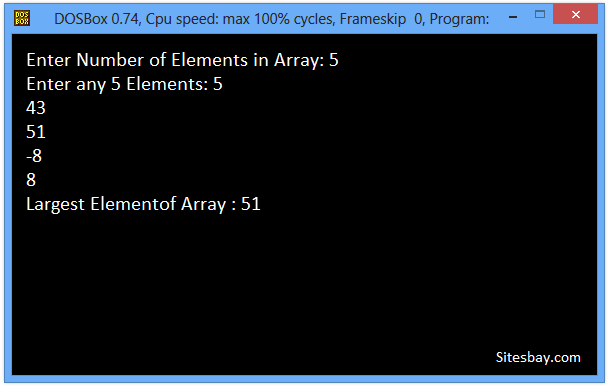# Find Largest Element of an Array Program in C++

## C++ Program to Find Largest Element of an Array

In this code, we will find the Largest element of array by using linear search. Given an array of N elements, we have to find the Largest element of array. In below C++ program we first take number of elements in array as input from user as store it in variable count

## Example

```Array : [21, 32, 51, -5, -4, 9, 0, -2]
Largest Elementof Array : 51
```

To understand below example, you have must knowledge of following C++ programming topics; String in C++, for looping statements we need know For Loop in C++ and Array in C++.

### Algorithm to Find Largest Element of Array

• First Take Number of elements as input from user. Let it be N.
• Then ask user to enter N numbers and store it in an array(lets call it input).
• Initialize one variables max with first element of input.
• Using a loop, traverse input from index 0 to N -1 and compare each element with max.
• If current element is less than max, then update max with current element.
• After array traversal max will have the Largest element.

## C++ Program to Find Largest Element of an Array

```#include<iostream.h>
#include<conio.h>

void main()
{

int input, count, i, max;

cout<<"Enter Number of Elements in Array\n";
cin>>count;

cout<<"Enter any "<<count<<" Elements \n";

for(i = 0; i < count; i++){
cin>>input[i];
}

max = input;
// search num in inputArray from index 0 to elementCount-1
for(i = 0; i < count; i++){
if(input[i] > max){
max = input[i];
}
}

cout<<"Maximum Element of Array\n"<<max;
getch();

}
```

## Output

```Enter Number of Elements in Array: 5
Enter any 5 Elements: 5
43
51
-8
8
Largest Elementof Array : 51
```## Pure VPN Privide Lowest Price VPN Just @ \$1.65. Per Month with Non Detected IP Lowest Price Non Detected IP VPN